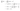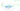## Gradient Descent for Linear Regression

The gradient descent algorithm can be used to minimize the cost function $J(\theta_0, \theta_1)$ for linear regression. We can substitute our actual cost function and our actual hypothesis function and modify the equation.

The value of $\frac{\partial}{\partial \theta_j} J(\theta)$ can be calculated as follows:When specifically applied to the case of linear regression, a new form of the gradient descent equation can be derived.

repeat until convergence: {

$\,\,\,\,\,\, \theta_0 := \theta_0 - \alpha \frac{1}{m} \sum\limits_{i=1}^{m}(h_\theta(x^{(i)}) - y^{(i)})$

$\,\,\,\,\,\, \theta_1 := \theta_1 - \alpha \frac{1}{m} \sum\limits_{i=1}^{m}\left((h_\theta(x^{(i)}) - y^{(i)}) x^{(i)}\right)$

}

In general, the gradient descent equation can be written as:

repeat until convergence: {

$\,\,\,\,\,\, \theta_j := \theta_j - \alpha \frac{1}{m} \sum\limits_{i=1}^{m}\left((h_\theta(x^{(i)}) - y^{(i)}) x^{(i)}_{j}\right) \,\,\,$ (simultaneously update $\theta_j$ every $j$)

}

If we start with a guess for our hypothesis (usually with $\theta_0 = 0$ and $\theta_1 = 0$) and then repeatedly apply these gradient descent equations, our hypothesis will become more and more accurate.

This method looks at every example in the entire training set on every step, and is called batch gradient descent. Note that, while gradient descent can be susceptible to local minima in general, the optimization problem we have posed here for linear regression has only one global, and no other local, optima; thus gradient descent always converges (assuming the learning rate α is not too large) to the global minimum. Indeed, J is a convex quadratic function.